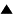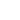The Principle of Causality# The Principle of Causality

 equal causes have equal effects (1)
This proposition is to be understood in both directions: "equal effects have (are the results of) equal causes" applies as well.

The former expression denotes predictability: if we know the effect e1 of c1, and we know that another cause c2 is equal to c1, then we can predict that c2 will have an effect e2 equal to e1. The latter (converse) expression denotes reversibility: if we know two equal effects e2 and e1, and we know that e1 was caused by c1, then we can retrodict the cause c2 equal to c1 of e2, that is to say we can reverse the process (at least informationally). The whole expression can also be reformulated as :

 distinct causes have distinct effects (and vice-versa). (2)

This expression can be summarized as distinction conservation: if two situations were initially distinct they will remain distinct in all further evolutions, and have been distinct during all previous evolutions.

An equivalent principle defining causality is that of covariation:

 when a cause is varied (replaced by a cause different in some respect), its associated effect will vary (3)

The problem with these different definitions is that if they are considered at the most fundamental, microscopic level, they become trivial, unable to produce any practical predictions. In (1), if "equal" is taken to mean "identic" we get the tautologous result that if a cause is identic to itself (which it always is), then its effect must be identic to itself (which can be seen as a paraphrase of the principle of the identity of the indistinguishables). In (2) or (3) we get that if an initial state is replaced by a state that is not identically the same, then the resulting state will not be identically the same. If the state is a "state of the world", encompassing all that exists, then it is obvious that after a change of that state the resulting state will not be identically the same as the one resulting before the change. (Otherwise we would not be able to retrodictively distinguish the two initial states, since no difference whatsoever would be left).

We may call such a radical interpretation of (1) and (2) the "microscopic causality" principle. This principle does not allow us to make any predictions. In practice, "equal" is therefore interpreted as "similar": two causes or events may be different in some way, yet behave the same as far as the things we are interested in are concerned.

However, recent advances in non-linear dynamics have shown that in general dynamic systems are chaotic, in the sense that small differences in initial conditions can lead to enormous differences in later conditions, so that any "similarity" between two initial states is lost. In such cases the causality principle becomes practically meaningless.

For example, consider a coin vertically resting on its side in an unstable equilibrium. When it falls, we will not look for the cause of it falling down this side rather than that side, but rather consider it a chance event. The reason is that the coin in an unstable equilibrium is so sensitive that it will covary with the tiniest variation in its neighbourhood: a little bit of wind, a vibration of the table, an irregularity in the surface, or even a random distribution of air molecules so that a little bit more molecules bump against one side rather than against the other. The coin follows the microscopic causality principle: the slightest difference in initial conditions makes a difference in results.

In practice, the causality principles mentioned above only make sense when equality or distinction can be interpreted macroscopically, by means of boundaries between equivalence classes of causes and effects, so that microscopic differences between causes within one equivalence class can be ignored and only the macroscopic differences between the classes need to be taken into account. This is "macroscopic causality". However, macroscopic causality will only work in certain cases (e.g. when the dynamics is non-chaotic), and therefore cannot be seen as a universal principle.

The classical world view can be characterized by the assumption that macroscopic causality, or more generally distinction conservation, is always valid. The non-classical view, which appears in quantum mechanics and far-from-equilibrium thermodynamics, is then characterized by distinction non-conservation. That means that initially distinct states may lead to the same result (distinction destruction), or that the same state might lead to distinct outcomes (distinction creation). This requires more general principles of evolution and self-organization, based on variation and selection. The fact that macroscopic causality itself works in certain cases may be explained by these principles.

Reference: Heylighen F. (1989):"Causality as Distinction Conservation: a theory of predictability, reversibility and time order", Cybernetics and Systems 20, p. 361-384.

 HomeMetasystem Transition TheoryPrinciples of Systems and Cybernetics Up Prev.Next Down